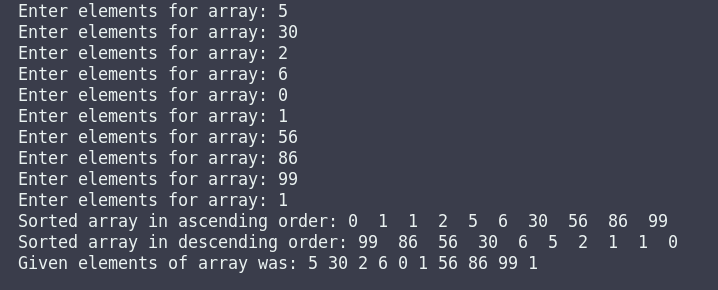# cpp programming exercise with solution

Write a c++ program in which you have to initialize create a function of any name and initialize an array of size ten into function and take input ten numbers from the user then print them into ascending and descending order to call function into the main function and also print the actual array after printing ascending and descending order.

Note: the main function should contain only function calls, not more.

Sample Program:

Enter elements for array: 5

Enter elements for array: 30

Enter elements for array: 2

Enter elements for array: 6

Enter elements for array: 0

Enter elements for array: 1

Enter elements for array: 56

Enter elements for array: 86

Enter elements for array: 99

Enter elements for array: 1

Sorted array in ascending order: 0  1  1  2  5  6  30  56  86  99

Sorted array in descending order: 99  86  56  30  6  5  2  1  1  0

Given elements of array was: 5 30 2 6 0 1 56 86 99 1

#include <iostream>
using namespace std;

void ArraySorting ()
{
int i = 0, j = 0, temp;
int arr;
int tempArray;
for (i = 0; i < 10; i++)
{
cout<<"Enter elements for array: ";
cin>>arr[i];
tempArray[i] = arr[i];
}

for (i = 0; i < 10; i++)
{
for (j = i+1; j < 10; j++)
{
if(arr[i] > arr[j])
{
temp = arr[i];
arr[i] = arr[j];
arr[j] = temp;
}
}
}

cout<<"Sorted array in ascending order: ";
for (i = 0 ; i < 10; i++)
{
cout<<arr[i]<<" ";
}
cout<<endl;

for (i = 0; i < 10; i++)
{
for (j = i+1; j < 10; j++)
{
if(arr[i] < arr[j])
{
temp = arr[i];
arr[i] = arr[j];
arr[j] = temp;
}
}
}
cout<<"Sorted array in descending order: ";
for (i = 0 ; i < 10; i++)
{
cout<<arr[i]<<" ";
}
cout<<endl;

cout<<"Given elements of array was: ";
for (i = 0; i < 10; i++)
{
cout<<tempArray[i]<<" ";
}
}

int main()
{
ArraySorting();

return 0;
}cpp programming exercise with solution